Past Year Questions: Highway Materials

# Past Year Questions: Highway Materials - Notes | Study Transportation Engineering - Civil Engineering (CE)

 1 Crore+ students have signed up on EduRev. Have you?

Question 1. If the fineness modulus of a sample of fine aggregates is 4.3, the mean size of the particles in the sample is between     [2019 : 1 Mark, Set-II]
(a) 300 μm and 600 μm
(b) 2.36 mm and 4.75 mm
(c) 1.18 mm and 2.36 mm
(d) 150 μm and 300 μm
(c)
Solution:
The sieves that are to be used for the sieve analysis of the aggregate (coarse, fine, or all- in-aggregate) for concrete as per IS:2386 (Part-1) -1963 are, 80 mm, 40 mm, 20 mm, 10 mm, 4.75 mm, 2.36 mm, 1.18 mm, 600 μm, 300 μm and 150 μm.
The fineness modulus can be regarded as a weighted average size of a sieve on which material is retained and the sieves being counted from the first sieve.
Fineness modulus of 4.3 indicates size between 4th and 5th sieve i.e., between 1.18 mm and 2.36 mm.

Question 2.The initial concavity in the Load-penetration curve of a CBR test is NOT due to    [2018 : 1 Mark, Set-II]
(a) uneven top surface
(c) inclined penetration plunger
(d) soft top layer of soaked soil
(b)
Solution:

Initial concavity in CBR test is due to :
• Improper compaction
• Soft top layer
• Inclined plunger
• Uneven top serface

Question 3. A bitumen sample has been graded as VG30 as per IS: 73-2013. The ‘30’ in the grade means that    [2018 : 1 Mark, Set-I]
(a) penetration of bitumen at 25°C is between 20 and 40
(b) viscosity of bitumen at 60°C is between 2400 and 3600 Poise
(c) ductility of bitumen at 27°C is more than 30 cm
(d) elastic recovery of bitumen at 15°C is more than 30%
(b)

Question 4. The following observations are made while testing aggregate for its suitability in pavement construction:
(i) Mass of oven-dry aggregate in air = 1000g
(ii) Mass of saturated surface-dry aggregate in air = 1025 g
(iii) Mass of saturated surface-dry aggregate underwater = 625 g Based on the above observations, the correct statement is    [2017 : 2 Marks, Set-I]
(a) bulk specific gravity of aggregate = 2.5 and water absorption = 2.5%
(b) bulk specific gravity of aggregate = 2.5 and water absorption = 2.4%
(c) apparent specific gravity of aggregate = 2.5 and water absorption = 2.5% (d) apparent specific gravity of aggregate = 2.5 and water absorption = 2.4%

Solution: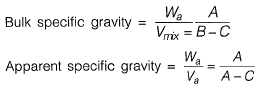Where,
A = Mass of oven dry aggregate in air
B = Mass of Saturated surface dry aggregate in air
C = Mass of Saturated surface dry aggregate underwater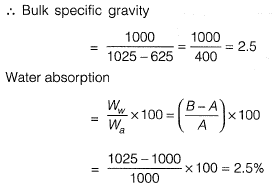Question 5. During a forensic investigation of pavement failure, an engineer reconstructed the graphs P, Q, R and S, using partial and damaged old reports.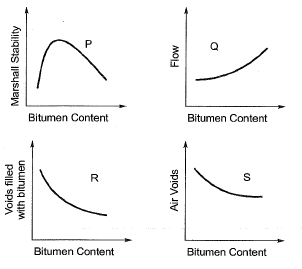Theoretically plausible correct graphs according to the ' Marshall mixture design output’ are   [2016 : 1 Mark, Set-I]
(a) P, Q, R
(b) P, Q, S
(c) Q, R, S
(d) R, S, P

Solution: The graph between VFB and Bitumen content is,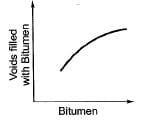Question 6. Match the information related to test on aggregates given in Group-I with that in Group-ll.   [2015 : 2 Marks, Set-II]
Group-I
P. Resistance to impact
Q. Resistance to wear
R. Resistance to weathering action
S. Resistance to crushing
Group-ll
1. Hardness
2. Strength
3. Toughness
4. Soundness
(a) P-1, Q-3, R-4, S-2
(b) P-3, Q-1, R-4, S-2
(c) P-4, Q-1, R-3, S-2
(d) P-3, Q-4, R-2, S-1

Question 7.  In Marshall method of mix design, the coarse aggregate, fine aggregate, fines and bitumen having respective values of specific gravity 2.60, 2.70, 2.65 and 1.01, are mixed in the relative proportions (% by weight) of 55.0, 35.8, 3.7 and 5.5 respectively. The theoretical specific gravity of the mix and the effective specific gravity of the aggregates in the mix respectively are    [2015 : 2 Marks, Set-II]
(a) 2.42 and 2.63
(b) 2.42 and 2.78
(c) 2.42 and 2.93
(d) 2.64 and 2.78
(a)
Solution: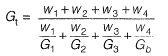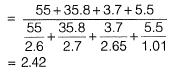Effective specific gravity of aggregates (coarse + fine) is given by,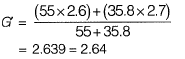Question 8. The penetration value of a bitumen sample tested at 25°C is 80. When this sample is heated to 60°C and tested again, the needle of the penetration test apparatus penetrates the bitumen sample by d mm. The value of d CANNOT be less than _________ mm.    [2015 : 1 Mark, Set-I]
Solution:
Relationship between penetration and temperature is given by,
P= AT+ K
Where P = Penetration
A = Temperature susceptibility
K= Constant
With in crease in temperature , penetration increases.
Hence, we will simply take,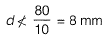Question 9. In a Marshall sample, the bulk specific gravity of mix and aggregates are 2.324 and 2.546 respectively. The sample includes 5% of bitumen (by total weight of mix) of specific gravity 1.10. The theoretical maximum specific gravity of mix is 2.441. The void filled with bitumen (VFB) in the Marshall sample (in %) i s ______ .     [2014 : 2 Marks, Set-II]
Solution: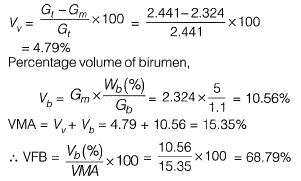Question 10. In compacted cylindrical bituminous mix
VMA = 15% (void mineral aggregate)
Vv = 4.5% (air void) The magnitude of VFB (void filled bituminous) is    [2013 : 2 Marks]
(a) 24
(b) 30
(c) 54
(d) 70
Solution: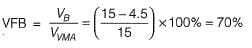Question 11. Two bitumen samples “A" and “ V” have softening points 45°C and 60°C, respectively. Consider the following statement:
I. Viscosity of “X” will be higher than that of “ V” at the same temperature.
II. Penetration value of “X ’ will be lesser than that of “ Y' under standard conditions.
The CORRECT option evaluating the above statements is      [2012 : 1 Mark]
(a) Both I and II are TRUE
(b) I is FALSE and II is TRUE
(c) Both I and II are FALSE
(d) I is TRUE and II is FALSE

Solution: Since softening point for Vis higher than X.
∴ Viscosity of Vis high as compared to Xand penetration will be more in X, because it will offer less resistance to penetrate.
∴ Both statement I & II are false.

Question 12. In Marshall testing of bituminous mixes, as the bitumen content increases the flow value    [2011 : 1 Mark]
(a) remains constant
(b) decreases first and then increases
(c) increases monotonically
(d) increases first and then decreases
Solution: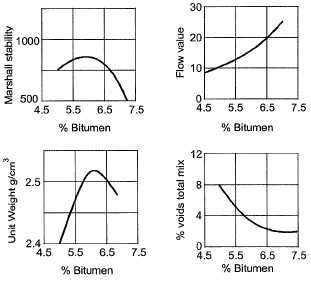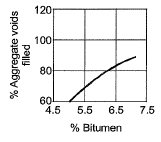Question 13. Aggregate impact value indicates which one of the following property of aggregates?    [2010 : 1 Mark]
(a) Durability
(b) Toughness
(c) Hardness
(d) Strength

The document Past Year Questions: Highway Materials - Notes | Study Transportation Engineering - Civil Engineering (CE) is a part of the Civil Engineering (CE) Course Transportation Engineering.
All you need of Civil Engineering (CE) at this link: Civil Engineering (CE)

## Transportation Engineering

22 videos|68 docs|39 tests
 Use Code STAYHOME200 and get INR 200 additional OFF

## Transportation Engineering

22 videos|68 docs|39 tests

Track your progress, build streaks, highlight & save important lessons and more!

,

,

,

,

,

,

,

,

,

,

,

,

,

,

,

,

,

,

,

,

,

;